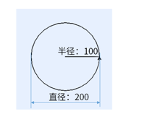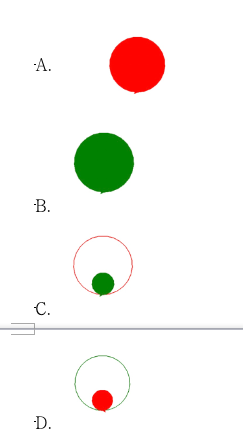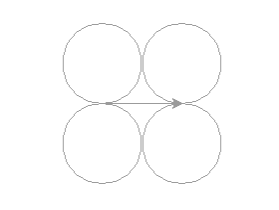# 2021年12月电子学会Python级别试卷（1级）答案解析1. 昨天的温度是5摄氏度，今天降温7摄氏度，今天的温度是多少摄氏度？（ ）

A. 12

B. 7

C. 2

D. -2

2.

x1="11"

x2="12"

x3= x1 + x2

print(x3)

x4 = int(x1) + int(x2)

print(x4)

A. 程序执行输出的x3与x4均为数值数据

B. print（x3）表示的含义为：打印整数相加

C. print（x4）表示的含义为：打印字符串相加

D. 程序执行输出x3为：1112，x4为：23

3. 编写一个程序，实现下面的功能：输入1个三位数时，自动会输出这个三位数的十位上的数字。小明已经写好了程序，但是由于不小心把第二行程序给删掉了，第一行代码是number=int(input("请输入一个三位数")) ，最后一行的代码分别是print(number_2)。请您帮小明选择下面哪个程序代码作为中间一行代码才是正确的？（ ）

A. number_2=(number-number//100*100)//10

B. number_2=(number-number%100*100)%10

C. number_2=(number-number**100*100)**10

D. number_2=(number-number/100*100)/10

4. 已知a=2,b=3,赋值语句a*=b+5执行后，a的值是多少？（ ）

A. 11

B. 16

C. 13

D. 26

5.

A. turtle.dot()

B. turtle.dot('red',50)

C. dot(50)

D. turtle.dot(50,'red')

6.A. 100*2

B. 100

C. 50

D. 200

7. 已知a = 4，b = 7那么print(a!=b)的结果为？（ ）

A. 4

B. 7

C. False

D. True

8.

a = input('输入一个整数')

b = a * 4

print(b)

A. 24

B. 6

C. 6666

D. 4

9. print(21//4)的结果是？（ ）

A. 5

B. 1

C. 4

D. 5.25

10. turtle.clear()命令的作用是？（ ）

A. 清空turtle窗口，但是turtle的位置和状态不会改变;

B. 清空turtle窗口，turtle的位置和状态会初始化;

C. 清空turtle中的变量，但是turtle的位置和状态不会改变;

D. 清空turtle中的变量，turtle的位置和状态会初始化;

11. 下列代码的执行结果是？（ ）

a=2

b=3

a=5

print (a**b-b/2*10)

A. 110.0

B. 110

C. 49.0

D. 49

12. 下列代码执行会报错的选项是？（ ）

A. a='6'

c=1+eval(a)

print(c)

B. a=24

c='10'+str(a)

print(c)

C. b=6

c=a+b

print(c)

D. a='ha'

c=a*2

print(c)

13.

①圆括号内的项目

②幂运算和根运算

③乘法和除法

④加法和减法

A. ①②③④

B. ②③④①

C. ③④①②

D. ④①②③

14. type() 函数返回对象的类型，那么print(type("354>324"))输出的结果是？（ ）

A. <class 'str'>

B. <class 'int'>

C. <class 'float'>

D. <class 'bool'>

15. 在turtle库中的指令，一般可以用turtle.speed(speed)来设置画笔移动速度，画笔绘制的速度范围是整数。以下正确的速度范围是？（ ）

A. 0~5的整数

B. 0~10的整数

C. 0~100的整数

D. 0~20的整数

16. 以下哪个符号不可能作为Python单行或多行注释使用的基本符号？（ ）

A. #

B. '''

C. *

D. """

17. 下列关于Python程序设计语言的说法，错误的是？（ ）

A. Python语法简洁、清晰，并且具有丰富和强大的类库

B. Python是一种面向对象、解释型计算机程序设计语言

C. Python只能在Windows操作系统上使用

D. Python的源程序以.py为扩展名

18. 执行print('"10+20="10+20')后，结果等于？（ ）

A. 10+20=30

B. "10+20"=30

C. 报错

D. "10+20="10+20

19. Python数据类型，下列说法不正确的是？（ ）

A. int可以转换为float型

B. float型不能转换为int型

C. int代表的是整型

D. type()函数可以查看变量的数据类型

20. 下面哪个字符不能用做变量名？（ ）

A. the

B. is

C. yes

D. no

21.

import turtle

turtle.color('green')

turtle.circle(80)

turtle.color('red')

turtle.begin_fill()

turtle.circle(30)

turtle.end_fill()22. print(1<2 and 3<2 or 3<4 and 'a'!='a' or not 2+3>=6)的结果是？（ ）

A. False

B. True

C. 2

D. 1

23. Turtle库中，直接跳转到（x,y）点的命令是？（ ）

A. turtle.go(x,y)

B. turtle.setup(y,x)

C. turtle.goto(x,y)

D. turtle.forward(x,y)

24. Turtle库中，用于设置画笔的颜色的命令是？（ ）

A. turtle.speed()

B. turtle.pencolor()

C. turtle.penup()

D. turtle.pensize()

25. 以下哪个是Python保存文件时的文件扩展名？（ ）

A. .yp

B. .py

C. .ppy

D. .yyp

26. 变量名大小写是没有区分的，如变量名stuname和StuName是没区别的。（ ）

27.

Python可以同一行显示多条语句，方法是在语句中间用分号“；”分开。（ ）

28.

import turtle

turtle.penup()

turtle.color('green')

turtle.circle(50)

turtle.pendown()

29. 在Python中输入80/4//3,计算结果为6.0。（ ）

30. turtle库中，我们用turtle.setup()函数来设置画布大小，参数width,height为小于等于1.0的小数时，表示的是宽和高占据电脑屏幕的比例。

31. 输入print(7//2),输出结果为3.0。（ ）

32.

Python语言会要求缩进，但是不严格缩进，也不影响代码运行。（ ）

Python语言会严格要求缩进，如果不按照规定缩进，可能会出现语法错误甚至逻辑错误。

33. 在Python中，对输入的数学比较运算式进行判断，当输入"3>=2"时（注：输入的内容不包含双引号），Python运行的结果是“True”。（ ）

34.

IDLE的交互式编辑窗口和脚本式编辑窗口之间的主要外观区别之一是：脚本式有">>>"命令执行提示符，而交互式没有。（ ）

35. 在安装了Python环境的PC机上，可以在命令窗口中执行C:>python script.py来运行python程序（script.py为文件名）。（ ）

36. 描述

第一行输入一个数字，代表爸爸的身高

第二行输入一个数字，代表妈妈的身高

第三行输入一个数字（1或-1），代表性别系数

（输入使用input()，并有相应提示信息）

输出孩子未来的身高。

（输出使用print()，并有相应提示信息）

程序仅供参考，结果符合题意即可。

参考程序如下：

f=input("请输入爸爸的身高:")

m=input("请输入妈妈的身高:")

s=input("请输入性别系数:")

f=float(f)

m=float(m)

s=float(s)

h=(f+m+13*s)/2

print("孩子未来的身高:",h,"(厘米)")

（1）有输入语句并且有清楚的提示字符串；(每个一分，共3分）

（2）有输出语句并且按照题意输出；(2分）

（3）有类型转化语句；(2分）

（4）有计算语句；(2分）

（5）程序运行结果符合题目要求。(1分）

37.

（1）海龟的初始位置的坐标为（0，0）；

（2）绘制第1个左上方的圆形，半径为40；

（3）绘制第2个左下方的圆形，半径为40；

（4）海龟由初始位置坐标（0，0）移动至坐标（80，0）；

（5）绘制第3个右上方的圆形，半径为40；

（6）绘制第4个右下方的圆形，半径为40；import turtle

turtle.circle(40)

turtle.circle(-40)

turtle.forward(80)

turtle.circle(40)

turtle.circle(-40)

turtle.done()

（1）海龟初始位置在画布中心（2分）

（2）可以正确绘制第1个左上方的圆（4分）

（3）可以正确绘制第2个左下方的圆（4分）

（4）海龟移动到正确的位置（2分）

（5）可以正确绘制第3个右上方的圆（4分）

（6）可以正确绘制第4个右下方的圆（4分）